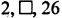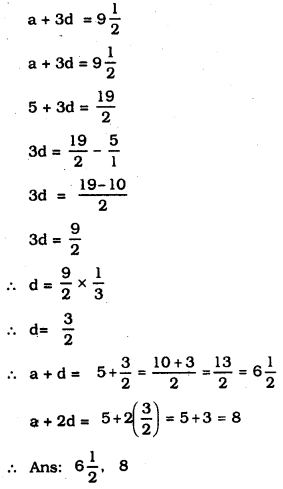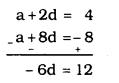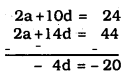# KSEEB SSLC Class 10 Maths Solutions Chapter 1 Arithmetic Progressions Ex 1.2

In this chapter, we provide KSEEB SSLC Class 10 Maths Solutions Chapter 1 Arithmetic Progressions Ex 1.2 for English medium students, Which will very helpful for every student in their exams. Students can download the latest KSEEB SSLC Class 10 Maths Solutions Chapter 1 Arithmetic Progressions Ex 1.2 pdf, free KSEEB SSLC Class 10 Maths Solutions Chapter 1 Arithmetic Progressions Ex 1.2 book pdf download. Now you will get step by step solution to each question.

### Karnataka State Syllabus Class 10 Maths Chapter 1 Arithmetic Progressions Ex 1.2

Question 1.
Fill in the blanks In the following table,
given that ‘a’ is the first term, ‘d’ is the common difference and an the nth term of the A.P.Solution:
(i) a = 7, d = 3, n = 8, an =?
an = a + (n – 1) d
a8= 7 + (8 – 1) 3
= 7 + 7 × 3
= 7 + 21
∴ a8 = 28

(ii) a = -18, d =?, n = 10, an = 0
an = a + (n – 1) d
0 = -18 + (10 – 1) d
0 = -18 + 9d
18 = 9d
9d = 18
d=189=2

(iii) a =?, d = -3, n = 18, an = -5
an = a + (n – 1) d
-5 = a + (18 – 1) (-3)
= a + 17(-3)
-6 = 1 – 51
∴ a = -5 + 51 = 46

(iv) a = -18.9, d = 2.5, n =? an = 3.6
an = a + (n – 1) d
3.6= -18.9 + (n – 1) (2.5)
3.6= -18.9 + 2.5n – 2.5
3.6 = 2.5n – 21.4
2.5n = 3.6 + 21.4
2.5n = 25
n=252.5=25025
∴ n = 10.

(v) a = 3.5, d = 0, n = 105, an =?
an = a + (n – 1) d
= 3.5 + (105 – 1) (0)
= 3.5+ 104 × 0
= 3.5 +0
∴ an = 3.5

Question 2.
Choose the correct choice In the following and justify:
(i) 30th term of the AP: 10, 7, 4, ……….. is
A) 97
B) 77
C) -77
D) -87
Solution:
a = 10. d = 7 – 10 = -3, n = 30, a30 =?
an = a + (n – 1) d
a30 = 10 + (30 – 1)(-3)
= 10 + 29(-3)
= 10 – 87
∴ a30 = 77
∴ Ans: (C) -77

(ii) 11th term of the A.P.
−3,−12,2,……, is
A) 28
B) 22
C) -38
D) −4812
Solution:
a=−3,d=−12−(−3)=−12+3=212
n = 11, a11 =?
an = a + (n – 1) d
a11=−3+(11−1)(212)
=−3+10(52)
= -3 +25
∴ a11 = 22
∴ Ans: (B) 22

Question 3.
In the following APs find the missing terms in the boxes:Solution:
(i)a = 2, a + d =?, a + 2d = 26
a + 26 = 26
2 + 2d = 26
2d = 24
∴ d=12 . .
∴ a + d = 2 + 12 = 14
∴ Ans: 14

(ii) Here, a =?, a + d = 13, a + 2d=?,
a + 3d = 3
a + d + 2d = 3
13 + 2d = 3
2d = 3 – 13
2d = -10
∴ d = -5
a + d = 13
a + (-5) = 13
a – 5 = 13
∴ a – 13 + 5 = 18
∴ a = 18
a + 2d =?
= 18 + 2(-5)
= 18 – 10
a + 2d = 8
∴ Ans: 18, 8

(iii) a = 5, a + d =?, a + 2d =?(iv) a = -4, a +d=? a + 2d =?
a+3d = ? a + 4d =? a + 5d = 6
a + 5d = 6
-4 + 5d = 6
5d = 6 + 4
5d = 10
d=105
∴ d=2.
a + d = -4 + 2 = -2
a + 2d = -4 + 2 (2) = -4 + 4 = 0
a + 3d = -4 + 3 (2) = -4 + 6 = 2
a + 4d = -4 + 4 (2) = -4 + 8 = 4
∴ Ans: -2, 0, 2, 4

(v) a =? a + d = 38, a + 2d =?
a + 3d =?, a + 4d =?a + 5d = 224d = -60
d=−−604=−15
a + d = 38
a – 15=38
a = 38 + 15 = 53
a + 2d = 53 + 2(-15) = 53 – 30 = 23
a + 3d = 53 + 3(-15) = 53 – 45 = 8
a + 4d =53 + 4(-15) = 53 – 60 = 7
∴ 53, 23, 8, -7.

Question 4.
Which term of the AP : 3, 8, 13. 18, ………. is 78?
Solution:
a = 3, d = 8 – 3 = 5, an = 78, n =?
an = a + (n – 1) d
78 = 3+(n—1)(5)
78 = 3 + 5n – 5
78 = 5n – 2
5n = 78 + 2
n=805
∴ n = 16

Question 5.
Find the number of terms In each of the following APs:
(i) 7, 13, 19, ………… 201
(ii) 18,1512,13,……47
Solution:
(i) 7, 13, 19, ………. 201
a = 3, d = 13 – 7 = 6, an = 201. n =?
a + (n – 1) d = an
3 + (n – 1) 6 = 201
3 + 6n – 6 = 201
6n – 3 = 201
6n = 203
n=2046
∴ n = 34

(ii) 18,1512,13,……47
a=18,d=a2−a1=31−362=−52
an = -47, n =?
an = a + (n – 1) d
−47=18+(n−1)(−52)
−47−18=(n−1)(−52)
(n−1)(−52)=−65
n−1=−65×−25
n – 1 = -13 × -2
n – 1 = + 26
∴ n = 26 + 1
∴ n = 27

Question 6.
Check whether -150 is a term of the A.P: 11, 8, 5, 2, ………..
Solution:
11, 8, 5, 2, ……….. -150
a = 11, d = 8 11 = -3. an = -150.
a + (n – 1) d = an
11 + (n – 1) (-3) = -150
11 – 3n + 3 = -150
-3n + 14 = -150
-3n = -150 – 14
-3n = -164
3n = 164
n=1643
Here value of ‘n’ is not perfect. Hence -150 is not a term of the A.P.

Question 7.
Find the 31st term of an AP whose 11th term Is 38 and the 16th term is 73.
Solution:
a = 38, a16 = 83 a31 =?
an = a + (n – 1) d
a16 = a + (16 – 1) d
a + 15d = 83
38 + 15d = 83
15d = 83 – 38
15d = 45
d=4515
∴ d=3.
∴ an = a + (n – 1)d
a31 = 38 + (31 – 1) 3
= 38 + 30 × 3
= 38 + 90
∴ a = 128.

Question 8.
An AP consists of 50 terms of which 3rd term is 12 and the last term is 106. Find the 29th term.
Solution:
n = 50, a3 = 12, an = 106, a29 =?
a11 = a + (n – 1) d
a50 = a + (50 – 1) d = 106
∴ a + 49d = 106 ……………… (1)
a= a + 2d = 12 ………………. (2)
Subtracting equation (2) in equation (1).Substituting the value of d.
a + 2d = 12
a + 2(2) = 12
a + 4 = 12
∴ a = 12 – 4
a = 8.
∴ an = a + (n – 1) d
a29 = 8 + (29 – 1) 2
= 8 + 28 × 2
= 8 + 56
∴ a29 = 64

Question 9.
If the 3rd and the 9th terms of an AP are 4 and -8 respectively, which term of this AP is zero?
Solution:
a3 = 4, a9 = -8, an = 0, n =?
a3 = a + 2d = 4 ………….. (1)
a9 = a + 8d = -8 …………. (2)
From equation (1) – equation (2).6d = 12
d=−126
∴ d = -2
a + 2d = 4
a – 2(2) = 4
a – 4 =4
∴ a = 4 + 4
∴ a = 8
an = a + (n – 1) d
= 8 + (n – 1) (-2)
= 8 – 2n + 2
= 10 – 2n = 0 ∵ an = 0
n=102
∴ n = 5
∴ 5th term of this AP is Zero.

Question 10.
The 1 7th term of an AP exceeds its 17th term by 7. Find the common difference.
Solution:
a17 = a10 + 7, d =?
a + 16d = a + 9d + 7
a +1 6d – a – 9d = 7
7d = 7
d=77
∴ = 1

Question 11.
Which term of the AP: 3, 15, 27. 39, … will be 132 more than its 54th term?
Solution:
3, 15, 27, 39, ………… an =?, n =?
an = a54 + 132
a = 3, d = 15 – 3 = 12
an = a54 + 132
an = a + 53d + 132
3 + 53(12) + 132
= 3 + 636 + 132
∴ an = 771
an = a + (n – 1) d = 771
= 3 + (n – 1)12 = 771
3 + 12n — 12 = 771
12n – 9 = 771
12n = 771 + 9
12n = 780
n=78012
∴ n = 65.
∴ 65th term is 132 more than its 54th term.

Question 12.
Two APs have the same common difference. The difference between their 100th terms is 100. what is the difference between their 1 000th terms?
Solution:
Having common difference ‘d’, the APs
1st set a, a+d, a+2d
2nd set b, b + d, b + 2d
100th term of 1st set – 100th term of 2nd set = 100
∴ a + 99d – (b + 99d) = 100
a + 99d – b – 99d = 100
a – b= 100
Similarly.
1000th term of 1st set = 1 + 999d
1000 th term of 2nd set = b + 999d
Their difference
= a + 999d – (b + 999d)
= a + 999d – b – 999d
= a – b.

Question 13.
How many three-digit numbers are divisible by 7?
Solution:
First three-digit number divisible by 7
105 and the List number Is 994.
∴ AP is 105, 112. 119 994.
a = 105, d = 112 – 105 = 7. an = 994.
n =?
a + (n – 1)d = an
105 + (n – 1) 7 = 994
105 + 7n – 7 = 994
7n + 98 = 994
7n = 994 – 98
7n = 896
n=8967
∴ n = 128.
∴ Numbers with 3 digits divisible by 7 are 128.

Question 14.
How many multiples of 4 lie between 10 and 250?
Solution:
Multiples of 4. after 10 are 12, 16, 20….
Multiples of 4 upto 250 is 248
∴ A.P. is 12, 16, 20, …….. 248
a = 12, d = 16 – 12 = 4
n =?
a = a + (n – 1) d = 248
12 + 4n – 4 = 248
4n + 8 = 248
4n = 248 – 8
4n = 240
n=2404
∴ n = 60
∴ Multiples of 4 lie between 10 and 250 is 60.

Question 15.
For what value of n’. are the nth terms of two APs: 63, 65, 67, ……. and 3, 10, 17, ……….. equal?
Solution;
63, 65, 67,……….
a = 63. d = 65 – 63 =2. an =?
nth term of this is
an = a + (n – 1)d
= 63 + (n – 1) 2
= 63 + 2n – 2
an= 2n + 61 …………….. (i)
3, 10, 17, ………….
a = 3, d = 10 – 3 = 7, an =?
an = a + (n – 1)d
= 3 + (n – 1) 7
= 3 + 7n – 7
an = 7n — 4 ………….(ii)
Here. nth terms of second AP are equal.
∴ equation (i) = equation (ii)
2n + 61 = 7n – 4
2n – 7n = -4 – 61
5n = 65
5n =65
n=655
∴ n = 13
∴13th terms of the two given APs are equal.

Question 16.
DetermIne the AP whose third term is 16 and 7th term exceeds the 5th term by 12.
Solution:
a = 16, a7 = a5 + 12, A.P =?
a7 = a5 + 12
a + 6d = a + 4d + 12
a + 6d – a – 4d = 12
2d = 12 ∴ d = 6.
a + 2d = 16
a + 2(6) = 16
a + 12 = 16
∴ a = 16 – 12 ∴ a = 4
a = 4, d = 6.
∴ A.P.:
a, a + d, a + 2d, …………………
4, 4 + 6, 4 + 12, …………….
4, 10, 16, ………….

Question 17
Find the 20th term from the Last term of theAP: 3, 8, 13, …………., 253.
Solution:
3, 8, 13, ………….., 253
a = 3. d = 8 – 3 = 5, an = 253
20th term from the last term of the AP starting from 253 =?
253, 258, 263, ………… a20 =?
a = 253, d = 258 – 253 = 5, n = 20
an = a + (n – 1) d
a20= 253 + (20 – 1) 5
= 253 + 19 × 5
= 253 + 95
∴ a20 = 348
∴ 20th term from the last term of the AP is 348.

Question 18.
The sum of the 4th and 8th terms of an AP is 24 and the sum of the 6th and 10th terms is 44. FInd the first three terms of the AP.
Solution:
a4 + a8 = 24 …………. (1)
a6 + a10 = 44 ………… (2)
But A.P is a, a + d, a + 2d
from equation (1).
a4 + a8 = 24
a + 3d + a + 7d = 24
2a + 10d = 24 ………….. (3)
from equation (2).
a6 + a10 = 44
a + 5d + a + 9d = 44
2a + 14d = 44 ………… (4)
Subtracting eqn. (4) from equation (3)4d = 20
d=20/4
∴ d = 5
Substituting the value of d in equation (3)
2a + 10d = 24
2a + 10(5) = 24
2a + 50 = 24
2a = 24 – 50
2a = -26
a = 26/2 ,
∴ a = -13 .
∴ AP: a, a + d, a + 2d, ………..
-13, -13 + 5, -13 + 2(5), ………
-14, -8, -3, …………

Question 19.
Subba Rao started work in 1995 at an annual salary of Rs. 5000 and received an Increment of Rs. 200 each year. in which year did his income reach Rs. 7000?
Solution:
Payment of Subba Rao in the year 1995 = Rs. 5000
increment = Rs. 200
∴ The payment he received in the year 1996 is Rs. 5.200a = 5000, d = 5200 – 5000 = 200,
an = 7000, n=?
a + (n – 1)d = an
5000 + (n – 1) 200 = 7000
5000 + 200n – 200 = 7000
200n + 4800 = 7000
200n = 7000 – 4800
200n = 2200
n=2200200
∴ n = 11
Value of ‘n’ is 11
∴ From 1995 to 10 years means 2005. his salary becomes Rs. 7,000.

Question 20.
Ramkall saved Rs. 5 In the first week of a year and then Increased her weekly savings by Rs. 1.75. If in the nüI week. her weekly savings become Rs. 20.75. find ‘n’.
Solution:
Savings in the First week = Rs. 5.
Savings in the Second week 5 + 1.75 = Rs. 6.75
Savings in ‘nth week is Rs. 20.75
∴ A.P. 5, 6, 75 , …………. , 20.75
a = 5, d = 6.75 – 5 = 1.75, an = 20.75.
n =?
a + (n – 1) d = an
5 + (n – 1) (1.75) = 20.75
5 + 1.75n – 1.75 = 20.75
1 .75n + 3.25 = 20.75
1.75n = 20.75 – 3.25
1.75n = 17.5
n=17.51.75
n=1750175
∴ n = 10
∴ In the 10th week, her savings becomes Rs. 20.75.

All Chapter KSEEB Solutions For Class 10 Maths

—————————————————————————–

All Subject KSEEB Solutions For Class 10

*************************************************

I think you got complete solutions for this chapter. If You have any queries regarding this chapter, please comment on the below section our subject teacher will answer you. We tried our best to give complete solutions so you got good marks in your exam.

If these solutions have helped you, you can also share kseebsolutionsfor.com to your friends.

Best of Luck!!